Test: Control System- 2

# Test: Control System- 2 - Electrical Engineering (EE)

Test Description

## 20 Questions MCQ Test GATE Electrical Engineering (EE) 2023 Mock Test Series - Test: Control System- 2

Test: Control System- 2 for Electrical Engineering (EE) 2023 is part of GATE Electrical Engineering (EE) 2023 Mock Test Series preparation. The Test: Control System- 2 questions and answers have been prepared according to the Electrical Engineering (EE) exam syllabus.The Test: Control System- 2 MCQs are made for Electrical Engineering (EE) 2023 Exam. Find important definitions, questions, notes, meanings, examples, exercises, MCQs and online tests for Test: Control System- 2 below.
Solutions of Test: Control System- 2 questions in English are available as part of our GATE Electrical Engineering (EE) 2023 Mock Test Series for Electrical Engineering (EE) & Test: Control System- 2 solutions in Hindi for GATE Electrical Engineering (EE) 2023 Mock Test Series course. Download more important topics, notes, lectures and mock test series for Electrical Engineering (EE) Exam by signing up for free. Attempt Test: Control System- 2 | 20 questions in 60 minutes | Mock test for Electrical Engineering (EE) preparation | Free important questions MCQ to study GATE Electrical Engineering (EE) 2023 Mock Test Series for Electrical Engineering (EE) Exam | Download free PDF with solutions
 1 Crore+ students have signed up on EduRev. Have you?
Test: Control System- 2 - Question 1

### With the value of "a" set for phase margin of π/4, the value of unit impulse response of the open loop system at t = 1 second is equal to

Test: Control System- 2 - Question 2

### The transfer function of a second order system is given as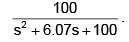The peak (A) value of the output for unit  step input is

Detailed Solution for Test: Control System- 2 - Question 2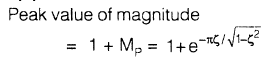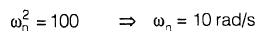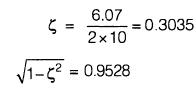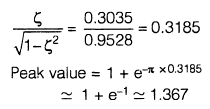Test: Control System- 2 - Question 3

### If the resonant peak of the system is B, then the value of B/A is

Detailed Solution for Test: Control System- 2 - Question 3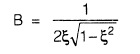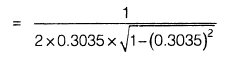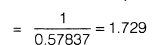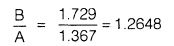Test: Control System- 2 - Question 4

A portion of the polar plot of an open-loop transfer function is shown in the given figure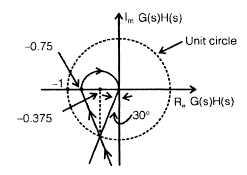The phase margin and gain margin will be respectively

Detailed Solution for Test: Control System- 2 - Question 4

Phase margin = 90° − 30° = 60° Gain margin = 1/0.75

Test: Control System- 2 - Question 5

The open-loop transfer function of a unity negative feedback system is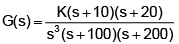The polar plot of the system will be

Detailed Solution for Test: Control System- 2 - Question 5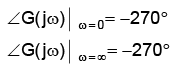The polar plot intersects with the negative real axis as in making imaginary term of G(jω) to be zero, the solution exists.

Test: Control System- 2 - Question 6

The compensator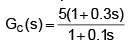would provide a maximum phase shift of

Detailed Solution for Test: Control System- 2 - Question 6

Maximum phase shift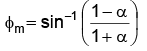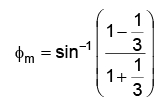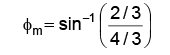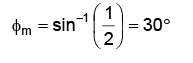Test: Control System- 2 - Question 7

A step input is applied to a system with the transfer function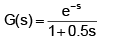The output response will be

Detailed Solution for Test: Control System- 2 - Question 7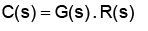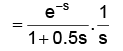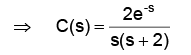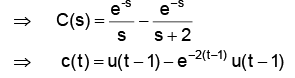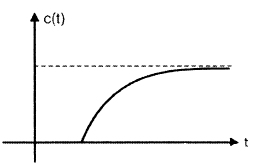Test: Control System- 2 - Question 8

Which one of the following characteristic equations of result in the stable operation of the feedback system?

Detailed Solution for Test: Control System- 2 - Question 8

For stable operation, all coefficients of the characteristic equation should be real and have the same sign and none of the coefficients should be zero.

Test: Control System- 2 - Question 9

Consider the following statements:
Routh-Hurwitz criterion gives
1. absolute stability
2. the number of roots lying on the right half of the s-plane
3. the gain margin and phase margin
Which of these statements are correct?

Which of these statements are correct?

Detailed Solution for Test: Control System- 2 - Question 9

Routh - Hurwitz criterion gives absolute stability and the number of roots lying on the right half of the s-plane but it does not tell about the gain margin and phase margin.

Test: Control System- 2 - Question 10

The polar plot (for positive frequencies) for the open-loop transfer function of a unity feedback control system is shown in the given figure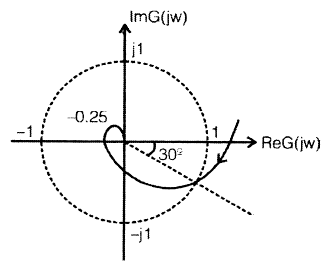The phase margin and the gain margin ofthe system are respectively

Detailed Solution for Test: Control System- 2 - Question 10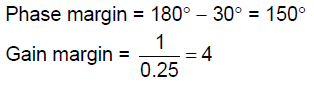Test: Control System- 2 - Question 11

For the given network, the maximum phase lead φm of V0 with respect to Vi is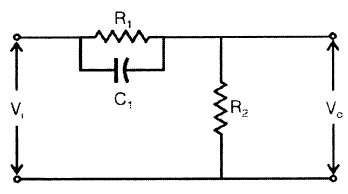Detailed Solution for Test: Control System- 2 - Question 11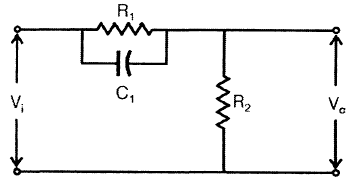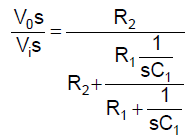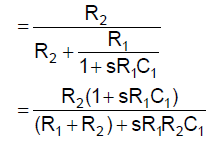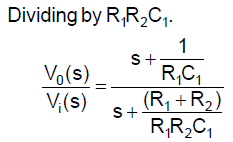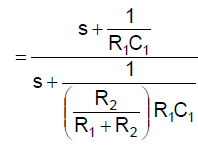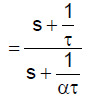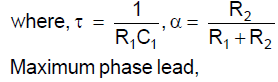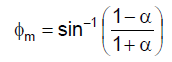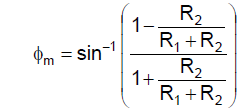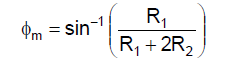Test: Control System- 2 - Question 12

For the system dynamics described by differential equationy+3y+2y=u(t) the transfer function of the system represented in controllable canonical form is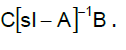The matrix A would be

Detailed Solution for Test: Control System- 2 - Question 12

General representation of phase variable representation: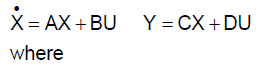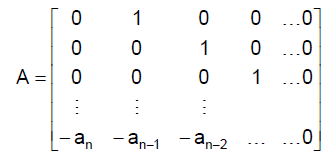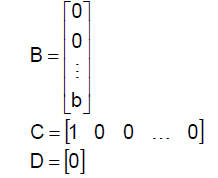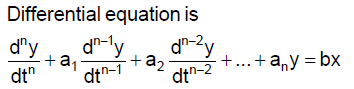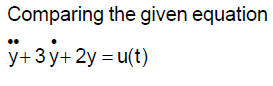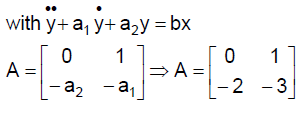Test: Control System- 2 - Question 13

For the signal flow diagram shown in the given figure, the transmittance between x2 and x1 is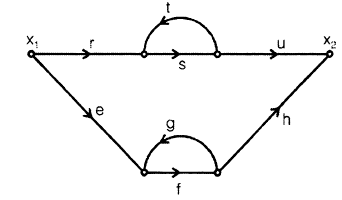Detailed Solution for Test: Control System- 2 - Question 13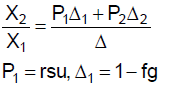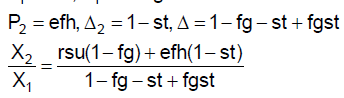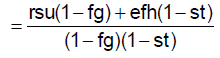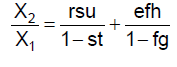Test: Control System- 2 - Question 14

Consider the mechanical system shown in the given figure. If the system is set into motion by unit impulse force, the equation of the resulting oscillation will be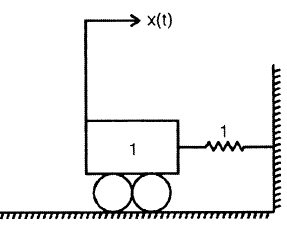Detailed Solution for Test: Control System- 2 - Question 14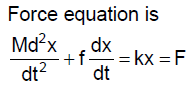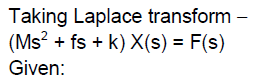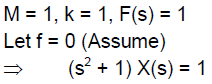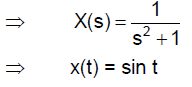Test: Control System- 2 - Question 15

Nyquist plot shown in the given figure is for a type

1.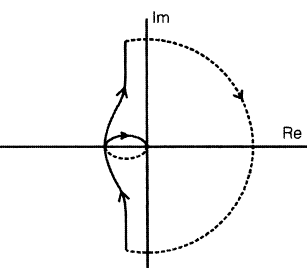Detailed Solution for Test: Control System- 2 - Question 15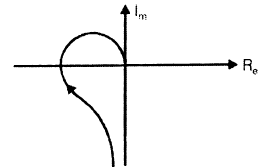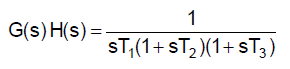(i) When a pole is added at negative real axis, the tail of the plot remains at the same position whereas head of plot shifts by 90° in clockwise direction.

(ii) When a pole is added at origin, both the tail and head of the plot shift by 90° in clockwise direction.

Test: Control System- 2 - Question 16

An open loop transfer function of a unity feedback control system has two finite zeros, two poles at origin and two pairs of complex conjugate poles. The slope of high frequency asymptote in Bode magnitude plot will be

Detailed Solution for Test: Control System- 2 - Question 16

No. of poles, n = 6
No. of zeros, m = 2
Slope of high frequency asymptote
= −20 (n−m)
= −20 (6−2)

Test: Control System- 2 - Question 17

Match List-I (Pole-zero plot of linear control system) with
List-II (Responses of the system) and select the correct answer:
Codes: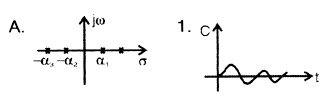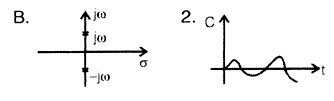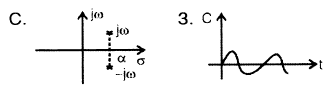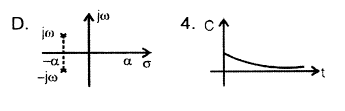Codes:

A    B    C  D

Test: Control System- 2 - Question 18

The unit impulse response of a system having transfer function K/(s + α) is shown below. The value of α is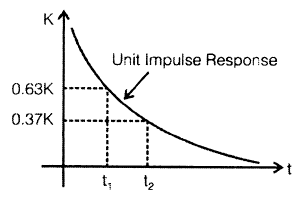Detailed Solution for Test: Control System- 2 - Question 18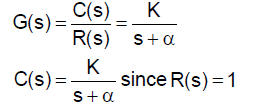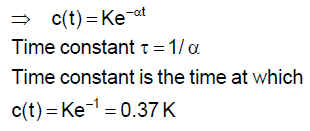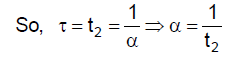Test: Control System- 2 - Question 19

The transfer function of a phase lead network as shown in the figure below is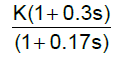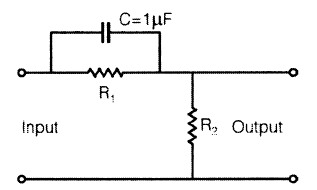The values of R1 and R2 are respectively

Detailed Solution for Test: Control System- 2 - Question 19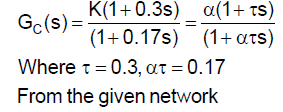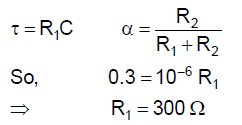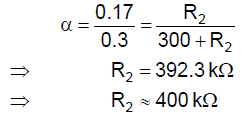Test: Control System- 2 - Question 20

The forward path transfer function of a unity feedback system is given by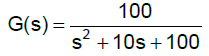The frequency response of this system will exhibit the resonance peak at

Detailed Solution for Test: Control System- 2 - Question 20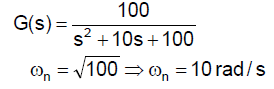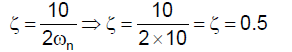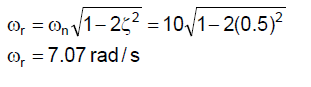## GATE Electrical Engineering (EE) 2023 Mock Test Series

22 docs|284 tests
Information about Test: Control System- 2 Page
In this test you can find the Exam questions for Test: Control System- 2 solved & explained in the simplest way possible. Besides giving Questions and answers for Test: Control System- 2, EduRev gives you an ample number of Online tests for practice

## GATE Electrical Engineering (EE) 2023 Mock Test Series

22 docs|284 tests(Scan QR code)